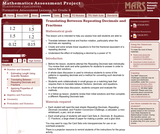Updating search results...

1 Result

View
Selected filters:
• MCCRS.Math.Content.8.NS.A.2Only Sharing Permitted
CC BY-NC-ND
Rating
4,0 stars

This lesson unit is intended to help teachers assess how well students are able to: translate between decimal and fraction notation, particularly when the decimals are repeating; create and solve simple linear equations to find the fractional equivalent of a repeating decimal; and understand the effect of multiplying a decimal by a power of 10.

نوع المادة:
التقييم
Lesson Plan
Provider:
Shell Center for Mathematical Education
Provider Set:
Mathematics Assessment Project (MAP)